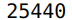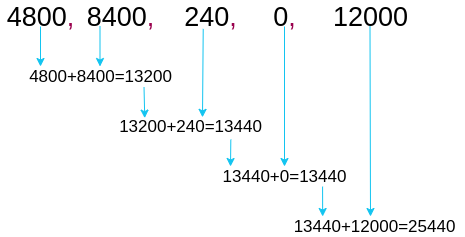Abhishek Sharma — Published On March 12, 2020 and Last Modified On April 26th, 2023

## Introduction

For loops are the antithesis of efficient programming. They’re still necessary and are the first conditional loops taught to Python beginners, but in my opinion, they leave a lot to be desired. These for loops can be cumbersome and can make our Python code bulky and untidy. But wait – what’s the alternative solution? Python Lambda Functions!

Lambda functions offer a dual boost to a data scientist. You can write tidier Python code and speed up your machine-learning tasks. The trick lies in mastering lambda functions, and this is where beginners can trip up. Initially, I also found lambda functions difficult to understand. They are short in length yet can appear confusing to a newcomer. But once I understood how to use them in Python, I found them very easy and powerful. And I’m sure you will as well by the end of this tutorial.

• In this python tutorial, you’ll be learning about the power of lambda functions in Python programming.
• You will learn how to use them with some built-in functions.
• You will also learn the difference between normal functions and lambda functions.

Note: New to Python? I highly recommend checking out the below free courses to get up to scratch:

## What Are Lambda Functions?

A lambda function is a small function containing a single expression written in a single line. Lambda functions can also act as anonymous functions where they don’t require function name or identifier. These are very helpful when we have to perform small tasks with less code.

We can also use lambda functions when we have to pass a small function to another function. Don’t worry – we’ll cover this in detail soon when we see how to use lambda functions in Python.

Lambda functions were first introduced by Alonzo Church in the 1930s. Mr. Church is well known for lambda calculus and the Church-Turing Thesis.

Lambda functions are handy and used in many programming languages, but we’ll be focusing on using them in Python here. In Python, lambda functions have the following syntax:

lambda y : x

here the lambda function takes argument y, evaluates it, and return x

Lambda functions consist of three parts:

• Lambda Keyword
• Bound variable/lambda argument, and
• Body or lambda expression

The keyword is mandatory, and it must be a lambda, whereas the arguments and body can change based on the requirements. You must be wondering why you should go for lambda functions when you have other regular functions. Fair question – let me elaborate on this.

## Comparing Lambda Functions With Regular Functions

Lambda functions are defined using the keyword lambda. They can have any number of arguments but only one expression. A lambda function cannot contain any statements, and it returns a function object which can be assigned to any variable. They are generally used for one-line expressions.

Normal functions are created using the def keyword. They can have any number of arguments, any number of expressions, and lines of code. They are generally used for large blocks of code.

## IIFEs Using Lambda Functions in Python

IIFEs are Immediately Invoked Function Expressions. These are functions that are executed as soon as they are created. IIFEs require no explicit call to invoke the function. In Python, IIFEs can be created using the lambda function.

Here, I have created an IIFE that returns the cube of a number.

Python Code:

Awesome!

## Application of Lambda Functions in Python

Time to jump into Python! Fire up your Jupyter Notebook, and let’s get to know some use cases of lambda functions.

We can use the lambda function with some built-in functions like the filter function to select certain items from iterables like lists, arrays, tuples, dictionaries, etc. Also, we can use lambda functions in a list comprehension.

Here, In the following example, I have created a random dataset that contains information about a family of 5 people with their id, names, ages, and income per month. I will be using this dataframe to show you how to apply lambda functions using different functions on a dataframe in Python.

Python Code:

#### Lambda With Apply

Let’s say we have an error in the age variable. We recorded ages with a difference of 3 years. So, to remove this error from the Pandas dataframe, we have to add three years to every person’s age. We can do this with the apply() function in Pandas.

apply() function calls the lambda function and applies it to every row or column of the dataframe and returns a modified copy of the dataframe:

``df['age']=df.apply(lambda x: x['age']+3,axis=1)``

We can use the apply() function to apply the lambda function to both rows and columns of a dataframe. If the axis argument in the apply() function is 0, then the lambda function gets applied to each column, and if 1, then the function gets applied to each row.

apply() function can also be applied directly to a Pandas series:

``df['age']=df['age'].apply(lambda x: x+3)``

In the above example, you can see that we got the same results using different methods.

#### Lambda With Filter Function

Now, let’s see how many of these people are above the age of 18. We can do this using the filter() function. The filter() function takes a lambda function and a Pandas series and applies the lambda function on the series, and filters the data, and a new list is returned, which contains all the items returned by that lambda function for each item.

This returns a sequence of True and False, which we use for filtering the data. Therefore, the input size of the map() function is always greater than the output size.

``list(filter(lambda x: x>18,df['age']))``

#### Lambda With Map Function

You’ll be able to relate to the next statement. It’s performance appraisal time, and the income of all the employees gets increased by 20%. This means we have to increase the salary of each person by 20% in our Pandas dataframe.

We can do this using the map() function. This map() function maps the series according to input correspondence. It is very helpful when we have to substitute a series with other values. In map() functions, the size of the input is equal to the size of the output.

``df['income']=list(map(lambda x: int(x+x*0.2),df['income']))``

#### Lambda With Reduce Function

Now, let’s see the total income of the family. To calculate this, we can use the reduce() function in Python. It is used to apply a particular function to the list of elements in the sequence. The reduce() function is defined in the ‘functools’ module.

For using the reduce() function, we have to import the functools module first:

``````import functools
functools.reduce(lambda a,b: a+b,df['income'])``````reduce() function applies the lambda function to the first two elements of the series and returns the result. Then, it stores that result and again applies the same lambda function to the result and the next element in the series. Thus, it reduces the series to a single value.

Note: Lambda functions in reduce() cannot take more than two arguments.

## Conditional Statements Using Lambda Functions

Lambda functions also support conditional statements, such as if..else. This makes lambda functions very powerful.

Let’s say in the family dataframe, we have to categorize people into ‘Adult’ or ‘Child.’ For this, we can simply apply the lambda function to our dataframe:

``df['category']=df['age'].apply(lambda x: 'Adult' if x>=18 else 'Child')``

Here, you can see that Ryan is the only child in this family, and the rest are adults. That wasn’t so difficult, was it?

## Conclusion

To summarize everything that has been stated so far, lambda functions are a powerful tool for creating simple, one-line functions in Python. They offer a concise and readable way to define functions without the need for a separate def statement. With the ability to create anonymous functions that can be passed as arguments to other functions, lambda functions can simplify code and make it more efficient. While they may not be appropriate for all situations, lambda functions can be a valuable addition to any Python programmer’s toolkit. By mastering lambda functions, you can write cleaner, more elegant code that is easier to understand and maintain.

Key Takeaways

• Lambda functions are used to define small, one-line functions in Python. They can be used for a wide range of tasks, including filtering, mapping, and sorting data.
• They are anonymous functions that can be used in other functions as arg or be returned as values.
• Lambda functions can make code more efficient by eliminating the need for a separate def statement.

Q1. When should I use lambda functions?

A. You can use lambda functions whenever you need a small, anonymous, and one-lined function.

Q2. Are lambda functions faster than regular functions in Python?

A. No, their performance is similar; however, lambda functions can be more efficient as there is no need for any def statement like normal functions.

Q3. Can I use lambda functions with higher-order functions?

A. Yes, you can use lambda functions with higher-order functions like filter, map, reduce, etc.###### Abhishek Sharma

He is a data science aficionado, who loves diving into data and generating insights from it. He is always ready for making machines to learn through code and writing technical blogs. His areas of interest include Machine Learning and Natural Language Processing still open for something new and exciting.